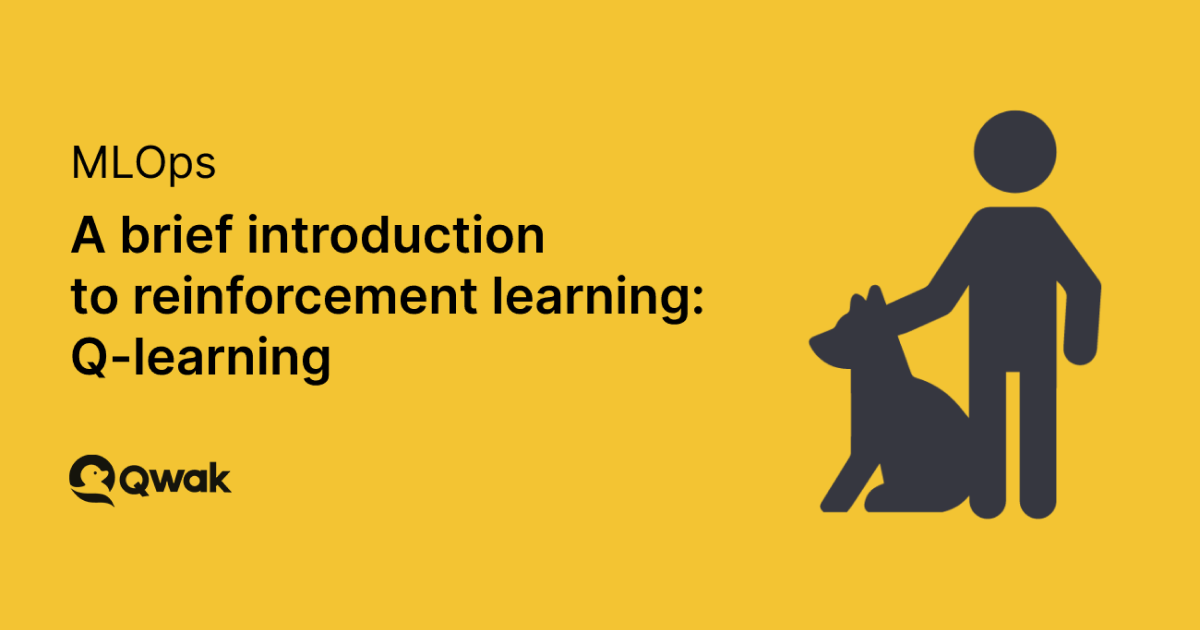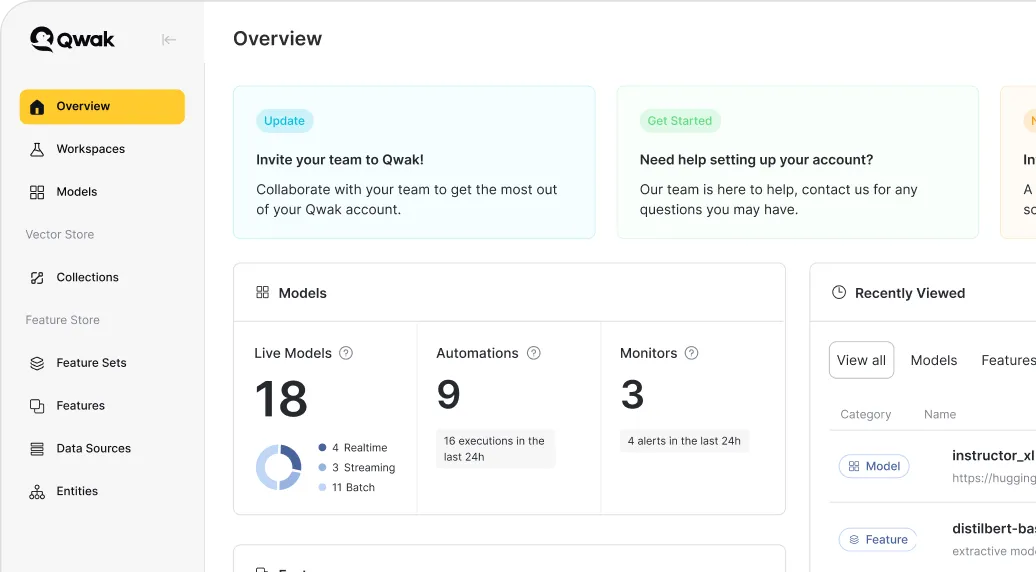# A brief introduction to reinforcement learning: Q-learning

Have you ever trained a pet to do something and rewarded it when it gets it right? This is known as reinforcement learning...Ran Romano
Co-founder & CPO at Qwak
May 26, 2022Have you ever trained a pet to do something and rewarded it when it gets it right? This is known as reinforcement learning, and it can be mirrored in machine learning to train a system to perform the desired action on command.

You have probably already heard of AI systems like AlphaGo and AlphaZero. These computer programs, developed by Deepmind Technologies, which is a subsidiary of Google, were developed to play the board game ‘Go’.

Go is known as the most challenging classical game for artificial intelligence because of its complexity. Despite decades of work, the strongest Go computer programs were only able to play at a similar level to human amateurs. Standard AI methods, which test all possible moves and positions using a search tree, were unable to handle the sheer number of possible Go moves or evaluate the strength of each possible board position.

So, when Deepmind’s AlphaGo became the first computer program to beat a professional human Go player in 2016, it understandably made headlines. At the same time, AlphaGo became the first computer program to defeat a Go world champion and arguably became the strongest Go player in history.

AlphaGo and programs like it are developed following reinforcement learning principles.

## What is reinforcement learning?

Reinforcement learning is an area of machine learning concerned with how intelligent agents ought to take actions in an environment to maximize the notion of cumulative reward.

In other words, it is the training of machine learning models to make a sequence of decisions. In reinforcement learning, the agent learns to achieve a goal in an uncertain and potentially complex environment, typically through a game-like situation, using trial and error to come up with a solution to the problem it is faced with.

To get the program to do what the programmer wants, it is rewarded or penalized for the actions it is performs, forcing the program to maximize its total reward by producing desired outcomes. While the programmer decides on the reward policy and the rules of the game, the model is not given any hints or suggestions for solving the problem; it is entirely up to the model to figure this out itself, starting with randomized trials and, hopefully, finishing with more sophisticated tactics.

## Basic reinforcement learning example

Let’s look at an example.

Imagine that you are trying to teach your dog a new trick. The dog doesn’t understand you, so you can’t tell it what to do. Instead, you need to create a situation (i.e., a visual cue) that the dog will be able to understand and respond to in different ways. If the dog’s response to the visual cue is the desired one, it will be rewarded with a snack.

Over time, the dog will form a connection between the cue and the action required to receive a reward, thus learning to carry out this action on command.

## When to use reinforcement learning in ML?

Although teaching a dog new tricks is great, the best and most powerful applications for reinforcement learning are found in AI and machine learning. More specifically, reinforcement learning is useful when you have got very little or no training data, or lack the expertise required to solve a particular problem.

On a higher level, your ML team will know what it wants to achieve but not how to get there. In this scenario, all you need is a reward mechanism in place and the reinforcement learning model will figure out for itself how to maximize the reward by solving the problem over time.

This is analogous to the example we gave above. If you want to teach a dog to sit down using treats, it will be clueless at first as it responds to your “Sit down!” command in various ways. At some point, it will sit down, and it will be rewarded because that’s the desired action. Iterate this scenario enough times and the dog will figure out how to receive a reward by sitting down on cue.

## The Q-learning algorithm

To teach programs using reinforcement learnings, ML teams have a variety of algorithms that they can use. One of the most popular is Q-learning, which is a value-based reinforcement learning algorithm used to find the optimal action-selection policy by using a Q function. Indeed, it is Q-learning that sits at the heart of the AlphaGo program discussed earlier.

Here’s a simple overview courtesy of freeCodeCamp

Let’s say that a robot must cross a maze to reach an endpoint. In its path are mines, and the robot can only move by a single tile at a time. If the robot hits a mine, it’s game over. The robot must reach the endpoint in the quickest possible time.

The reward system is as follows:

• One point is lost per square moved.
• If the robot hits a power square, it gains a point.
• If the robot hits a mine, it loses 100 points and the game ends.
• If the robot reaches the end, it gets 100 points.

To solve this problem, we turn to what is known as a ‘Q-Table’. This is a table that is used to calculate the maximum expected rewards for an action at each state. In other words, it’s a reference guide for the best action at each state.

There will be four numbers of actions at each non-edge tile. When a robot is at a state it has the option of either moving up or down or right or left. This can be modeled in the Q-able as follows:

Each Q-Table score will be the maximum expected future reward that the robot will get if it performs that action at that state. This is of course an iterative process and so the Q-Table must be improved at each iteration. This raises an obvious question: How do we calculate the values of the Q-Table? By using the Q-learning algorithm!

## The Q-learning algorithm

Here’s a look at the algorithm:

### The Q-function

The Q-function uses the Bellman equation and has two inputs: state (s) and action (a).

Using this equation, we get the values of Q for all cells in the Q-Table. To start off with, these will all be zero because this is an iterative process.

As we start to explore the environment, these values will update over time because the Q-function gives us better approximations b constantly updating the values in the table.

These updates are handled by the Q-learning algorithm:

Each yellow box is one step, and steps two through five are repeated over and over (iteration)n) until a good Q-Table has been built. Let’s look at these steps in more detail.

### Step 1: Build Q-Table

We will first build a Q-table. There are n columns, where n= number of actions. There are m rows, where m= number of states. As mentioned above, these values are initialized at zero. In the robot example, there are four actions (a=4) and five states (s=5), so the table will include four columns and five rows.

### Step 2 and Step 3: Choose action and perform action

These two steps are carried out for an undefined amount of time, meaning that the step runs until the training loop is stopped. An action (a) and a state (s) will be chosen based on the Q-Table, but, initially, every Q-value will be zero anyway.

In the beginning, the robot will explore the environment at random and choose random actions. This is because the robot does not yet know anything about the environment and so cannot make informed choices. During exploration, the robot progressively becomes more confident in estimating the Q-values.

For argument's sake, let’s say the robot moves right. The Q-values for being at the start and moving right can now be updated using the Bellman equation.

### Steps 4 and 5: Evaluation

Now that an action has been taken and an outcome has been observed, the function Q(s, a) must be updated.

• S is the State or observation
• A is the Action the agent takes
• R is the Reward from taking an Action
• t is the time step
• α is the Learning Rate
• λ is the discount factor which causes rewards to lose their value over time so that more immediate rewards are prioritized

Steps four and five will be repeated over and over until the learning is stopped. By following this, the Q-Table will be updated, and the robot will learn over time using the Q-learning method.

If you are feeling a little confused by all the information overload, we recommend reading Reinforcement Q-Learning from Scratch in Python with OpenAI by Learn Data Science. This is a comprehensive and in-depth look, whereas our article is meant to serve as a brief introduction.

Interested in learning about many of the other concepts that underpin modern machine learning? Check out our blog resources for our latest thoughts, guides, tutorials, and more.

Chat with us to see the platform live and discover how we can help simplify your ML journey.